# fsrnca

Feature selection using neighborhood component analysis for regression

## Syntax

``mdl = fsrnca(X,Y)``
``mdl = fsrnca(X,Y,Name,Value)``

## Description

example

``mdl = fsrnca(X,Y)` performs feature selection for regression using the predictors in `X` and responses in `Y`.`fsrnca` learns the feature weights by a diagonal adaptation of neighborhood component analysis (NCA) with regularization.`

example

``mdl = fsrnca(X,Y,Name,Value)` performs feature selection for regression with additional options specified by one or more name-value pair arguments.`

## Examples

collapse all

Generate toy data where the response variable depends on the 3rd, 9th, and 15th predictors.

```rng(0,'twister'); % For reproducibility N = 100; X = rand(N,20); y = 1 + X(:,3)*5 + sin(X(:,9)./X(:,15) + 0.25*randn(N,1));```

Fit the neighborhood component analysis model for regression.

`mdl = fsrnca(X,y,'Verbose',1,'Lambda',0.5/N);`
``` o Solver = LBFGS, HessianHistorySize = 15, LineSearchMethod = weakwolfe |====================================================================================================| | ITER | FUN VALUE | NORM GRAD | NORM STEP | CURV | GAMMA | ALPHA | ACCEPT | |====================================================================================================| | 0 | 1.636932e+00 | 3.688e-01 | 0.000e+00 | | 1.627e+00 | 0.000e+00 | YES | | 1 | 8.304833e-01 | 1.083e-01 | 2.449e+00 | OK | 9.194e+00 | 4.000e+00 | YES | | 2 | 7.548105e-01 | 1.341e-02 | 1.164e+00 | OK | 1.095e+01 | 1.000e+00 | YES | | 3 | 7.346997e-01 | 9.752e-03 | 6.383e-01 | OK | 2.979e+01 | 1.000e+00 | YES | | 4 | 7.053407e-01 | 1.605e-02 | 1.712e+00 | OK | 5.809e+01 | 1.000e+00 | YES | | 5 | 6.970502e-01 | 9.106e-03 | 8.818e-01 | OK | 6.223e+01 | 1.000e+00 | YES | | 6 | 6.952347e-01 | 5.522e-03 | 6.382e-01 | OK | 3.280e+01 | 1.000e+00 | YES | | 7 | 6.946302e-01 | 9.102e-04 | 1.952e-01 | OK | 3.380e+01 | 1.000e+00 | YES | | 8 | 6.945037e-01 | 6.557e-04 | 9.942e-02 | OK | 8.490e+01 | 1.000e+00 | YES | | 9 | 6.943908e-01 | 1.997e-04 | 1.756e-01 | OK | 1.124e+02 | 1.000e+00 | YES | | 10 | 6.943785e-01 | 3.478e-04 | 7.755e-02 | OK | 7.621e+01 | 1.000e+00 | YES | | 11 | 6.943728e-01 | 1.428e-04 | 3.416e-02 | OK | 3.649e+01 | 1.000e+00 | YES | | 12 | 6.943711e-01 | 1.128e-04 | 1.231e-02 | OK | 6.092e+01 | 1.000e+00 | YES | | 13 | 6.943688e-01 | 1.066e-04 | 2.326e-02 | OK | 9.319e+01 | 1.000e+00 | YES | | 14 | 6.943655e-01 | 9.324e-05 | 4.399e-02 | OK | 1.810e+02 | 1.000e+00 | YES | | 15 | 6.943603e-01 | 1.206e-04 | 8.823e-02 | OK | 4.609e+02 | 1.000e+00 | YES | | 16 | 6.943582e-01 | 1.701e-04 | 6.669e-02 | OK | 8.425e+01 | 5.000e-01 | YES | | 17 | 6.943552e-01 | 5.160e-05 | 6.473e-02 | OK | 8.832e+01 | 1.000e+00 | YES | | 18 | 6.943546e-01 | 2.477e-05 | 1.215e-02 | OK | 7.925e+01 | 1.000e+00 | YES | | 19 | 6.943546e-01 | 1.077e-05 | 6.086e-03 | OK | 1.378e+02 | 1.000e+00 | YES | |====================================================================================================| | ITER | FUN VALUE | NORM GRAD | NORM STEP | CURV | GAMMA | ALPHA | ACCEPT | |====================================================================================================| | 20 | 6.943545e-01 | 2.260e-05 | 4.071e-03 | OK | 5.856e+01 | 1.000e+00 | YES | | 21 | 6.943545e-01 | 4.250e-06 | 1.109e-03 | OK | 2.964e+01 | 1.000e+00 | YES | | 22 | 6.943545e-01 | 1.916e-06 | 8.356e-04 | OK | 8.649e+01 | 1.000e+00 | YES | | 23 | 6.943545e-01 | 1.083e-06 | 5.270e-04 | OK | 1.168e+02 | 1.000e+00 | YES | | 24 | 6.943545e-01 | 1.791e-06 | 2.673e-04 | OK | 4.016e+01 | 1.000e+00 | YES | | 25 | 6.943545e-01 | 2.596e-07 | 1.111e-04 | OK | 3.154e+01 | 1.000e+00 | YES | Infinity norm of the final gradient = 2.596e-07 Two norm of the final step = 1.111e-04, TolX = 1.000e-06 Relative infinity norm of the final gradient = 2.596e-07, TolFun = 1.000e-06 EXIT: Local minimum found. ```

Plot the selected features. The weights of the irrelevant features should be close to zero.

```figure() plot(mdl.FeatureWeights,'ro') grid on xlabel('Feature index') ylabel('Feature weight')```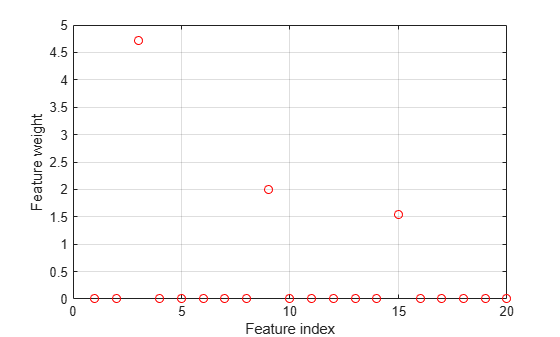`fsrnca` correctly detects the relevant predictors for this response.

`load robotarm.mat`

The `robotarm` (pumadyn32nm) dataset is created using a robot arm simulator with 7168 training observations and 1024 test observations with 32 features . This is a preprocessed version of the original data set. The data are preprocessed by subtracting off a linear regression fit, followed by normalization of all features to unit variance.

Perform neighborhood component analysis (NCA) feature selection for regression with the default $\lambda$ (regularization parameter) value.

```nca = fsrnca(Xtrain,ytrain,'FitMethod','exact', ... 'Solver','lbfgs');```

Plot the selected values.

```figure plot(nca.FeatureWeights,'ro') xlabel('Feature index') ylabel('Feature weight') grid on```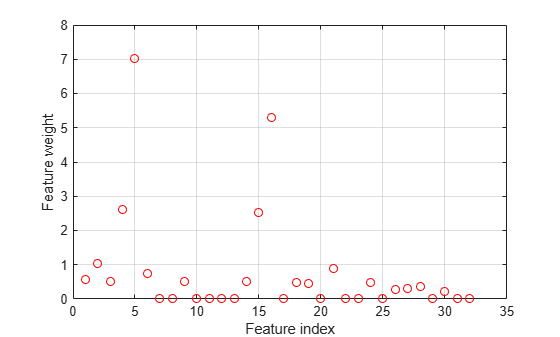More than half of the feature weights are nonzero. Compute the loss using the test set as a measure of the performance by using the selected features.

`L = loss(nca,Xtest,ytest)`
```L = 0.0837 ```

Try improving the performance. Tune the regularization parameter $\lambda$ for feature selection using five-fold cross-validation. Tuning $\lambda$ means finding the $\lambda$ value that produces the minimum regression loss. To tune $\lambda$ using cross-validation:

1. Partition the data into five folds. For each fold, `cvpartition` assigns 4/5th of the data as a training set, and 1/5th of the data as a test set.

```rng(1) % For reproducibility n = length(ytrain); cvp = cvpartition(length(ytrain),'kfold',5); numvalidsets = cvp.NumTestSets;```

Assign the $\lambda$ values for the search. Multiplying response values by a constant increases the loss function term by a factor of the constant. Therefore, including the `std(ytrain)` factor in the $\lambda$ values balances the default loss function (`'mad'`, mean absolute deviation) term and the regularization term in the objective function. In this example, the `std(ytrain)` factor is one because the loaded sample data is a preprocessed version of the original data set.

`lambdavals = linspace(0,50,20)*std(ytrain)/n;`

Create an array to store the loss values.

`lossvals = zeros(length(lambdavals),numvalidsets);`

2. Train the NCA model for each $\lambda$ value, using the training set in each fold.

3. Compute the regression loss for the corresponding test set in the fold using the NCA model. Record the loss value.

4. Repeat this for each $\lambda$ value and each fold.

```for i = 1:length(lambdavals) for k = 1:numvalidsets X = Xtrain(cvp.training(k),:); y = ytrain(cvp.training(k),:); Xvalid = Xtrain(cvp.test(k),:); yvalid = ytrain(cvp.test(k),:); nca = fsrnca(X,y,'FitMethod','exact', ... 'Solver','minibatch-lbfgs','Lambda',lambdavals(i), ... 'GradientTolerance',1e-4,'IterationLimit',30); lossvals(i,k) = loss(nca,Xvalid,yvalid,'LossFunction','mse'); end end```

Compute the average loss obtained from the folds for each $\lambda$ value.

`meanloss = mean(lossvals,2);`

Plot the mean loss versus the $\lambda$ values.

```figure plot(lambdavals,meanloss,'ro-') xlabel('Lambda') ylabel('Loss (MSE)') grid on```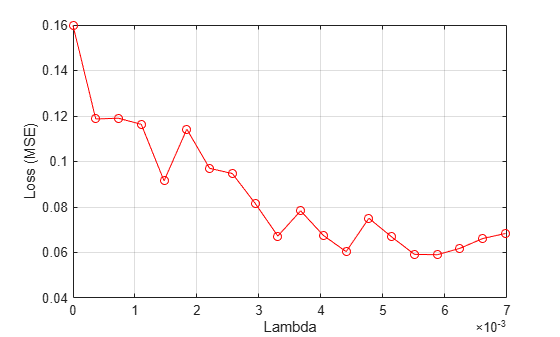Find the $\lambda$ value that gives the minimum loss value.

`[~,idx] = min(meanloss)`
```idx = 17 ```
`bestlambda = lambdavals(idx)`
```bestlambda = 0.0059 ```
`bestloss = meanloss(idx)`
```bestloss = 0.0590 ```

Fit the NCA feature selection model for regression using the best $\lambda$ value.

```nca = fsrnca(Xtrain,ytrain,'FitMethod','exact', ... 'Solver','lbfgs','Lambda',bestlambda);```

Plot the selected features.

```figure plot(nca.FeatureWeights,'ro') xlabel('Feature Index') ylabel('Feature Weight') grid on```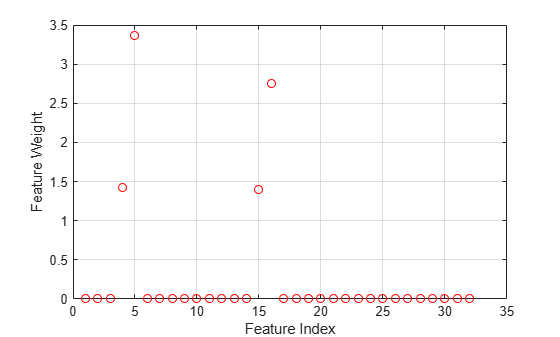Most of the feature weights are zero. `fsrnca` identifies the four most relevant features.

Compute the loss for the test set.

`L = loss(nca,Xtest,ytest)`
```L = 0.0571 ```

Tuning the regularization parameter, $\lambda$, eliminated more of the irrelevant features and improved the performance.

This example uses the Abalone data  from the UCI Machine Learning Repository .

Download the data and save it in your current folder with the name `'abalone.csv'`.

```url = 'https://archive.ics.uci.edu/ml/machine-learning-databases/abalone/abalone.data'; websave('abalone.csv',url);```

Read the data into a table. Display the first seven rows.

```tbl = readtable('abalone.csv','Filetype','text','ReadVariableNames',false); tbl.Properties.VariableNames = {'Sex','Length','Diameter','Height', ... 'WWeight','SWeight','VWeight','ShWeight','NoShellRings'}; tbl(1:7,:)```
```ans=7×9 table Sex Length Diameter Height WWeight SWeight VWeight ShWeight NoShellRings _____ ______ ________ ______ _______ _______ _______ ________ ____________ {'M'} 0.455 0.365 0.095 0.514 0.2245 0.101 0.15 15 {'M'} 0.35 0.265 0.09 0.2255 0.0995 0.0485 0.07 7 {'F'} 0.53 0.42 0.135 0.677 0.2565 0.1415 0.21 9 {'M'} 0.44 0.365 0.125 0.516 0.2155 0.114 0.155 10 {'I'} 0.33 0.255 0.08 0.205 0.0895 0.0395 0.055 7 {'I'} 0.425 0.3 0.095 0.3515 0.141 0.0775 0.12 8 {'F'} 0.53 0.415 0.15 0.7775 0.237 0.1415 0.33 20 ```

The dataset has 4177 observations. The goal is to predict the age of abalone from eight physical measurements. The last variable, the number of shell rings, shows the age of the abalone. The first predictor is a categorical variable. The last variable in the table is the response variable.

Prepare the predictor and response variables for `fsrnca`. The last column of `tbl` contains the number of shell rings, which is the response variable. The first predictor variable, sex, is categorical. You must create dummy variables.

```y = table2array(tbl(:,end)); X(:,1:3) = dummyvar(categorical(tbl.Sex)); X = [X,table2array(tbl(:,2:end-1))];```

Use four-fold cross-validation to tune the regularization parameter in the NCA model. First partition the data into four folds.

```rng('default') % For reproducibility n = length(y); cvp = cvpartition(n,'kfold',4); numtestsets = cvp.NumTestSets;```

`cvpartition` divides the data into four partitions (folds). In each fold, about three-fourths of the data is assigned as a training set and one-fourth is assigned as a test set.

Generate a variety of $\lambda$ (regularization parameter) values for fitting the model to determine the best $\lambda$ value. Create a vector to collect the loss values from each fit.

```lambdavals = linspace(0,25,20)*std(y)/n; lossvals = zeros(length(lambdavals),numtestsets);```

The rows of `lossvals` corresponds to the $\lambda$ values and the columns correspond to the folds.

Fit the NCA model for regression using `fsrnca` to the data from each fold using each $\lambda$ value. Compute the loss for each model using the test data from each fold.

```for i = 1:length(lambdavals) for k = 1:numtestsets Xtrain = X(cvp.training(k),:); ytrain = y(cvp.training(k),:); Xtest = X(cvp.test(k),:); ytest = y(cvp.test(k),:); nca = fsrnca(Xtrain,ytrain,'FitMethod','exact', ... 'Solver','lbfgs','Lambda',lambdavals(i),'Standardize',true); lossvals(i,k) = loss(nca,Xtest,ytest,'LossFunction','mse'); end end```

Compute the average loss for the folds, that is, compute the mean in the second dimension of `lossvals`.

`meanloss = mean(lossvals,2);`

Plot the $\lambda$ values versus the mean loss from the four folds.

```figure plot(lambdavals,meanloss,'ro-') xlabel('Lambda') ylabel('Loss (MSE)') grid on```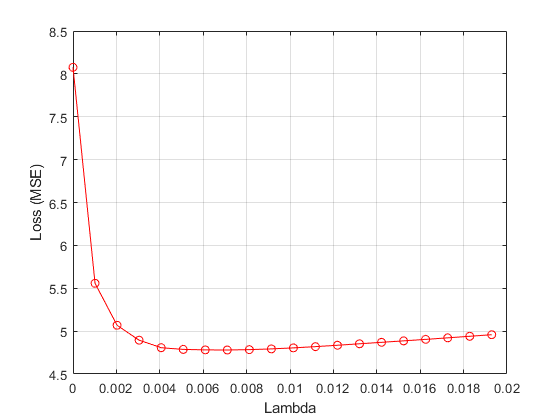Find the $\lambda$ value that minimizes the mean loss.

```[~,idx] = min(meanloss); bestlambda = lambdavals(idx)```
```bestlambda = 0.0071 ```

Compute the best loss value.

`bestloss = meanloss(idx)`
```bestloss = 4.7799 ```

Fit the NCA model on all of the data using the best $\lambda$ value.

```nca = fsrnca(X,y,'FitMethod','exact','Solver','lbfgs', ... 'Verbose',1,'Lambda',bestlambda,'Standardize',true);```
``` o Solver = LBFGS, HessianHistorySize = 15, LineSearchMethod = weakwolfe |====================================================================================================| | ITER | FUN VALUE | NORM GRAD | NORM STEP | CURV | GAMMA | ALPHA | ACCEPT | |====================================================================================================| | 0 | 2.469168e+00 | 1.266e-01 | 0.000e+00 | | 4.741e+00 | 0.000e+00 | YES | | 1 | 2.375166e+00 | 8.265e-02 | 7.268e-01 | OK | 1.054e+01 | 1.000e+00 | YES | | 2 | 2.293528e+00 | 2.067e-02 | 2.034e+00 | OK | 1.569e+01 | 1.000e+00 | YES | | 3 | 2.286703e+00 | 1.031e-02 | 3.158e-01 | OK | 2.213e+01 | 1.000e+00 | YES | | 4 | 2.279928e+00 | 2.023e-02 | 9.374e-01 | OK | 1.953e+01 | 1.000e+00 | YES | | 5 | 2.276258e+00 | 6.884e-03 | 2.497e-01 | OK | 1.439e+01 | 1.000e+00 | YES | | 6 | 2.274358e+00 | 1.792e-03 | 4.010e-01 | OK | 3.109e+01 | 1.000e+00 | YES | | 7 | 2.274105e+00 | 2.412e-03 | 2.399e-01 | OK | 3.557e+01 | 1.000e+00 | YES | | 8 | 2.274073e+00 | 1.459e-03 | 7.684e-02 | OK | 1.356e+01 | 1.000e+00 | YES | | 9 | 2.274050e+00 | 3.733e-04 | 3.797e-02 | OK | 1.725e+01 | 1.000e+00 | YES | | 10 | 2.274043e+00 | 2.750e-04 | 1.379e-02 | OK | 2.445e+01 | 1.000e+00 | YES | | 11 | 2.274027e+00 | 2.682e-04 | 5.701e-02 | OK | 7.386e+01 | 1.000e+00 | YES | | 12 | 2.274020e+00 | 1.712e-04 | 4.107e-02 | OK | 9.461e+01 | 1.000e+00 | YES | | 13 | 2.274014e+00 | 2.633e-04 | 6.720e-02 | OK | 7.469e+01 | 1.000e+00 | YES | | 14 | 2.274012e+00 | 9.818e-05 | 2.263e-02 | OK | 3.275e+01 | 1.000e+00 | YES | | 15 | 2.274012e+00 | 4.220e-05 | 6.188e-03 | OK | 2.799e+01 | 1.000e+00 | YES | | 16 | 2.274012e+00 | 2.859e-05 | 4.979e-03 | OK | 6.628e+01 | 1.000e+00 | YES | | 17 | 2.274011e+00 | 1.582e-05 | 6.767e-03 | OK | 1.439e+02 | 1.000e+00 | YES | | 18 | 2.274011e+00 | 7.623e-06 | 4.311e-03 | OK | 1.211e+02 | 1.000e+00 | YES | | 19 | 2.274011e+00 | 3.038e-06 | 2.528e-04 | OK | 1.798e+01 | 5.000e-01 | YES | |====================================================================================================| | ITER | FUN VALUE | NORM GRAD | NORM STEP | CURV | GAMMA | ALPHA | ACCEPT | |====================================================================================================| | 20 | 2.274011e+00 | 6.710e-07 | 2.325e-04 | OK | 2.721e+01 | 1.000e+00 | YES | Infinity norm of the final gradient = 6.710e-07 Two norm of the final step = 2.325e-04, TolX = 1.000e-06 Relative infinity norm of the final gradient = 6.710e-07, TolFun = 1.000e-06 EXIT: Local minimum found. ```

Plot the selected features.

```figure plot(nca.FeatureWeights,'ro') xlabel('Feature Index') ylabel('Feature Weight') grid on```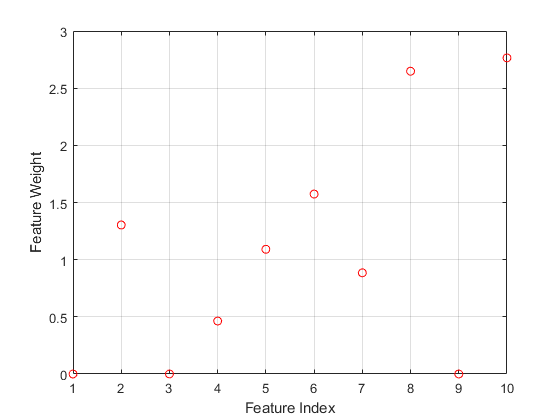The irrelevant features have zero weights. According to this figure, the features 1, 3, and 9 are not selected.

Fit a Gaussian process regression (GPR) model using the subset of regressors method for parameter estimation and the fully independent conditional method for prediction. Use the ARD squared exponential kernel function, which assigns an individual weight to each predictor. Standardize the predictors.

```gprMdl = fitrgp(tbl,'NoShellRings','KernelFunction','ardsquaredexponential', ... 'FitMethod','sr','PredictMethod','fic','Standardize',true)```
```gprMdl = RegressionGP PredictorNames: {'Sex' 'Length' 'Diameter' 'Height' 'WWeight' 'SWeight' 'VWeight' 'ShWeight'} ResponseName: 'NoShellRings' CategoricalPredictors: 1 ResponseTransform: 'none' NumObservations: 4177 KernelFunction: 'ARDSquaredExponential' KernelInformation: [1×1 struct] BasisFunction: 'Constant' Beta: 11.4959 Sigma: 2.0282 PredictorLocation: [10×1 double] PredictorScale: [10×1 double] Alpha: [1000×1 double] ActiveSetVectors: [1000×10 double] PredictMethod: 'FIC' ActiveSetSize: 1000 FitMethod: 'SR' ActiveSetMethod: 'Random' IsActiveSetVector: [4177×1 logical] LogLikelihood: -9.0019e+03 ActiveSetHistory: [1×1 struct] BCDInformation: [] Properties, Methods ```

Compute the regression loss on the training data (resubstitution loss) for the trained model.

`L = resubLoss(gprMdl)`
```L = 4.0306 ```

The smallest cross-validated loss using `fsrnca` is comparable to the loss obtained using a GPR model with an ARD kernel.

## Input Arguments

collapse all

Predictor variable values, specified as an n-by-p matrix, where n is the number of observations and p is the number of predictor variables.

Data Types: `single` | `double`

Response values, specified as a numeric real vector of length n, where n is the number of observations.

Data Types: `single` | `double`

### Name-Value Arguments

Specify optional pairs of arguments as `Name1=Value1,...,NameN=ValueN`, where `Name` is the argument name and `Value` is the corresponding value. Name-value arguments must appear after other arguments, but the order of the pairs does not matter.

Before R2021a, use commas to separate each name and value, and enclose `Name` in quotes.

Example: `'Solver','sgd','Weights',W,'Lambda',0.0003` specifies the solver as the stochastic gradient descent, the observation weights as the values in the vector `W`, and sets the regularization parameter at 0.0003.

Fitting Options

collapse all

Method for fitting the model, specified as the comma-separated pair consisting of `'FitMethod'` and one of the following:

• `'exact'` — Performs fitting using all of the data.

• `'none'` — No fitting. Use this option to evaluate the generalization error of the NCA model using the initial feature weights supplied in the call to fsrnca.

• `'average'` — Divides the data into partitions (subsets), fits each partition using the `exact` method, and returns the average of the feature weights. You can specify the number of partitions using the `NumPartitions` name-value pair argument.

Example: `'FitMethod','none'`

Number of partitions to split the data for using with `'FitMethod','average'` option, specified as the comma-separated pair consisting of `'NumPartitions'` and an integer value between 2 and n, where n is the number of observations.

Example: `'NumPartitions',15`

Data Types: `double` | `single`

Regularization parameter to prevent overfitting, specified as the comma-separated pair consisting of `'Lambda'` and a nonnegative scalar.

As the number of observations n increases, the chance of overfitting decreases and the required amount of regularization also decreases. See Tune Regularization Parameter in NCA for Regression to learn how to tune the regularization parameter.

Example: `'Lambda',0.002`

Data Types: `double` | `single`

Width of the kernel, specified as the comma-separated pair consisting of `'LengthScale'` and a positive real scalar.

A length scale value of 1 is sensible when all predictors are on the same scale. If the predictors in `X` are of very different magnitudes, then consider standardizing the predictor values using `'Standardize',true` and setting `'LengthScale',1`.

Example: `'LengthScale',1.5`

Data Types: `double` | `single`

Initial feature weights, specified as the comma-separated pair consisting of `'InitialFeatureWeights'` and a p-by-1 vector of real positive scalars, where p is the number of predictors in the training data.

The regularized objective function for optimizing feature weights is nonconvex. As a result, using different initial feature weights can give different results. Setting all initial feature weights to 1 generally works well, but in some cases, random initialization using `rand(p,1)` can give better quality solutions.

Data Types: `double` | `single`

Observation weights, specified as the comma-separated pair consisting of `'ObservationWeights'` and an n-by-1 vector of real positive scalars. Use observation weights to specify higher importance of some observations compared to others. The default weights assign equal importance to all observations.

Data Types: `double` | `single`

Indicator for standardizing the predictor data, specified as the comma-separated pair consisting of `'Standardize'` and either `false` or `true`. For more information, see Impact of Standardization.

Example: `'Standardize',true`

Data Types: `logical`

Verbosity level indicator for the convergence summary display, specified as the comma-separated pair consisting of `'Verbose'` and one of the following:

• 0 — No convergence summary

• 1 — Convergence summary, including norm of gradient and objective function values

• > 1 — More convergence information, depending on the fitting algorithm

When using `'minibatch-lbfgs'` solver and verbosity level > 1, the convergence information includes iteration the log from intermediate mini-batch LBFGS fits.

Example: `'Verbose',1`

Data Types: `double` | `single`

Solver type for estimating feature weights, specified as the comma-separated pair consisting of `'Solver'` and one of the following:

• `'lbfgs'` — Limited memory Broyden-Fletcher-Goldfarb-Shanno (LBFGS) algorithm

• `'sgd'` — Stochastic gradient descent (SGD) algorithm

• `'minibatch-lbfgs'` — Stochastic gradient descent with LBFGS algorithm applied to mini-batches

Default is `'lbfgs'` for n ≤ 1000, and `'sgd'` for n > 1000.

Example: `'solver','minibatch-lbfgs'`

Loss function, specified as the comma-separated pair consisting of `'LossFunction'` and one of the following:

• `'mad'` — Mean absolute deviation

`$l\left({y}_{i},{y}_{j}\right)=|{y}_{i}-{y}_{j}|.$`

• `'mse'` — Mean squared error

`$l\left({y}_{i},{y}_{j}\right)={\left({y}_{i}-{y}_{j}\right)}^{2}.$`

• `'epsiloninsensitive'` — ε-insensitive loss function

`$l\left({y}_{i},{y}_{j}\right)=\mathrm{max}\left(0,|{y}_{i}-{y}_{j}|-ϵ\right).$`

This loss function is more robust to outliers than mean squared error or mean absolute deviation.

• `@lossfun` — Custom loss function handle. A loss function has this form.

```function L = lossfun(Yu,Yv) % calculation of loss ...```
`Yu` is a u-by-1 vector and `Yv` is a v-by-1 vector. `L` is a u-by-v matrix of loss values such that `L(i,j)` is the loss value for `Yu(i)` and `Yv(j)`.

The objective function for minimization includes the loss function l(yi,yj) as follows:

`$f\left(w\right)=\frac{1}{n}\sum _{i=1}^{n}\sum _{j=1,j\ne i}^{n}{p}_{ij}l\left({y}_{i},{y}_{j}\right)+\lambda \sum _{r=1}^{p}{w}_{r}^{2},$`

where w is the feature weight vector, n is the number of observations, and p is the number of predictor variables. pij is the probability that xj is the reference point for xi. For details, see NCA Feature Selection for Regression.

Example: `'LossFunction',@lossfun`

Epsilon value for the `'LossFunction','epsiloninsensitive'` option, specified as the comma-separated pair consisting of `'LossFunction'` and a nonnegative real scalar. The default value is an estimate of the sample standard deviation using the interquartile range of the response variable.

Example: `'Epsilon',0.1`

Data Types: `double` | `single`

Memory size, in MB, to use for objective function and gradient computation, specified as the comma-separated pair consisting of `'CacheSize'` and an integer.

Example: `'CacheSize',1500MB`

Data Types: `double` | `single`

LBFGS Options

collapse all

Size of history buffer for Hessian approximation for the `'lbfgs'` solver, specified as the comma-separated pair consisting of `'HessianHistorySize'` and a positive integer. At each iteration the function uses the most recent `HessianHistorySize` iterations to build an approximation to the inverse Hessian.

Example: `'HessianHistorySize',20`

Data Types: `double` | `single`

Initial step size for the `'lbfgs'` solver, specified as the comma-separated pair consisting of `'InitialStepSize'` and a positive real scalar. By default, the function determines the initial step size automatically.

Data Types: `double` | `single`

Line search method, specified as the comma-separated pair consisting of `'LineSearchMethod'` and one of the following:

• `'weakwolfe'` — Weak Wolfe line search

• `'strongwolfe'` — Strong Wolfe line search

• `'backtracking'` — Backtracking line search

Example: `'LineSearchMethod','backtracking'`

Maximum number of line search iterations, specified as the comma-separated pair consisting of `'MaxLineSearchIterations'` and a positive integer.

Example: `'MaxLineSearchIterations',25`

Data Types: `double` | `single`

Relative convergence tolerance on the gradient norm for solver `lbfgs`, specified as the comma-separated pair consisting of `'GradientTolerance'` and a positive real scalar.

Example: `'GradientTolerance',0.000002`

Data Types: `double` | `single`

SGD Options

collapse all

Initial learning rate for the `'sgd'` solver, specified as the comma-separated pair consisting of `'InitialLearningRate'` and a positive real scalar.

When using solver type `'sgd'`, the learning rate decays over iterations starting with the value specified for `'InitialLearningRate'`.

The default `'auto'` means that the initial learning rate is determined using experiments on small subsets of data. Use the `NumTuningIterations` name-value pair argument to specify the number of iterations for automatically tuning the initial learning rate. Use the `TuningSubsetSize` name-value pair argument to specify the number of observations to use for automatically tuning the initial learning rate.

For solver type `'minibatch-lbfgs'`, you can set `'InitialLearningRate'` to a very high value. In this case, the function applies LBFGS to each mini-batch separately with initial feature weights from the previous mini-batch.

To make sure the chosen initial learning rate decreases the objective value with each iteration, plot the `Iteration` versus the `Objective` values saved in the `mdl.FitInfo` property.

You can use the `refit` method with `'InitialFeatureWeights'` equal to `mdl.FeatureWeights` to start from the current solution and run additional iterations

Example: `'InitialLearningRate',0.9`

Data Types: `double` | `single`

Number of observations to use in each batch for the `'sgd'` solver, specified as the comma-separated pair consisting of `'MiniBatchSize'` and a positive integer from 1 to n.

Example: `'MiniBatchSize',25`

Data Types: `double` | `single`

Maximum number of passes through all n observations for solver `'sgd'`, specified as the comma-separated pair consisting of `'PassLimit'` and a positive integer. Each pass through all of the data is called an epoch.

Example: `'PassLimit',10`

Data Types: `double` | `single`

Frequency of batches for displaying convergence summary for the `'sgd'` solver , specified as the comma-separated pair consisting of `'NumPrint'` and a positive integer. This argument applies when the `'Verbose'` value is greater than 0. `NumPrint` mini-batches are processed for each line of the convergence summary that is displayed on the command line.

Example: `'NumPrint',5`

Data Types: `double` | `single`

Number of tuning iterations for the `'sgd'` solver, specified as the comma-separated pair consisting of `'NumTuningIterations'` and a positive integer. This option is valid only for `'InitialLearningRate','auto'`.

Example: `'NumTuningIterations',15`

Data Types: `double` | `single`

Number of observations to use for tuning the initial learning rate, specified as the comma-separated pair consisting of `'TuningSubsetSize'` and a positive integer value from 1 to n. This option is valid only for `'InitialLearningRate','auto'`.

Example: `'TuningSubsetSize',25`

Data Types: `double` | `single`

SGD or LBFGS Options

collapse all

Maximum number of iterations, specified as the comma-separated pair consisting of `'IterationLimit'` and a positive integer. The default is 10000 for SGD and 1000 for LBFGS and mini-batch LBFGS.

Each pass through a batch is an iteration. Each pass through all of the data is an epoch. If the data is divided into k mini-batches, then every epoch is equivalent to k iterations.

Example: `'IterationLimit',250`

Data Types: `double` | `single`

Convergence tolerance on the step size, specified as the comma-separated pair consisting of `'StepTolerance'` and a positive real scalar. The `'lbfgs'` solver uses an absolute step tolerance, and the `'sgd'` solver uses a relative step tolerance.

Example: `'StepTolerance',0.000005`

Data Types: `double` | `single`

Mini-batch LBFGS Options

collapse all

Maximum number of iterations per mini-batch LBFGS step, specified as the comma-separated pair consisting of `'MiniBatchLBFGSIterations'` and a positive integer.

Example: `'MiniBatchLBFGSIterations',15`

Mini-batch LBFGS algorithm is a combination of SGD and LBFGS methods. Therefore, all of the name-value pair arguments that apply to SGD and LBFGS solvers also apply to the mini-batch LBFGS algorithm.

Data Types: `double` | `single`

## Output Arguments

collapse all

Neighborhood component analysis model for regression, returned as a `FeatureSelectionNCARegression` object.

 Rasmussen, C. E., R. M. Neal, G. E. Hinton, D. van Camp, M. Revow, Z. Ghahramani, R. Kustra, and R. Tibshirani. The DELVE Manual, 1996, https://mlg.eng.cam.ac.uk/pub/pdf/RasNeaHinetal96.pdf.

 University of Toronto, Computer Science Department. Delve Datasets. http://www.cs.toronto.edu/~delve/data/datasets.html.

 Nash, W.J., T. L. Sellers, S. R. Talbot, A. J. Cawthorn, and W. B. Ford. "The Population Biology of Abalone (Haliotis species) in Tasmania. I. Blacklip Abalone (H. rubra) from the North Coast and Islands of Bass Strait." Sea Fisheries Division, Technical Report No. 48, 1994.

 Waugh, S. "Extending and Benchmarking Cascade-Correlation: Extensions to the Cascade-Correlation Architecture and Benchmarking of Feed-forward Supervised Artificial Neural Networks." University of Tasmania Department of Computer Science thesis, 1995.

 Lichman, M. UCI Machine Learning Repository. Irvine, CA: University of California, School of Information and Computer Science, 2013. http://archive.ics.uci.edu/ml.